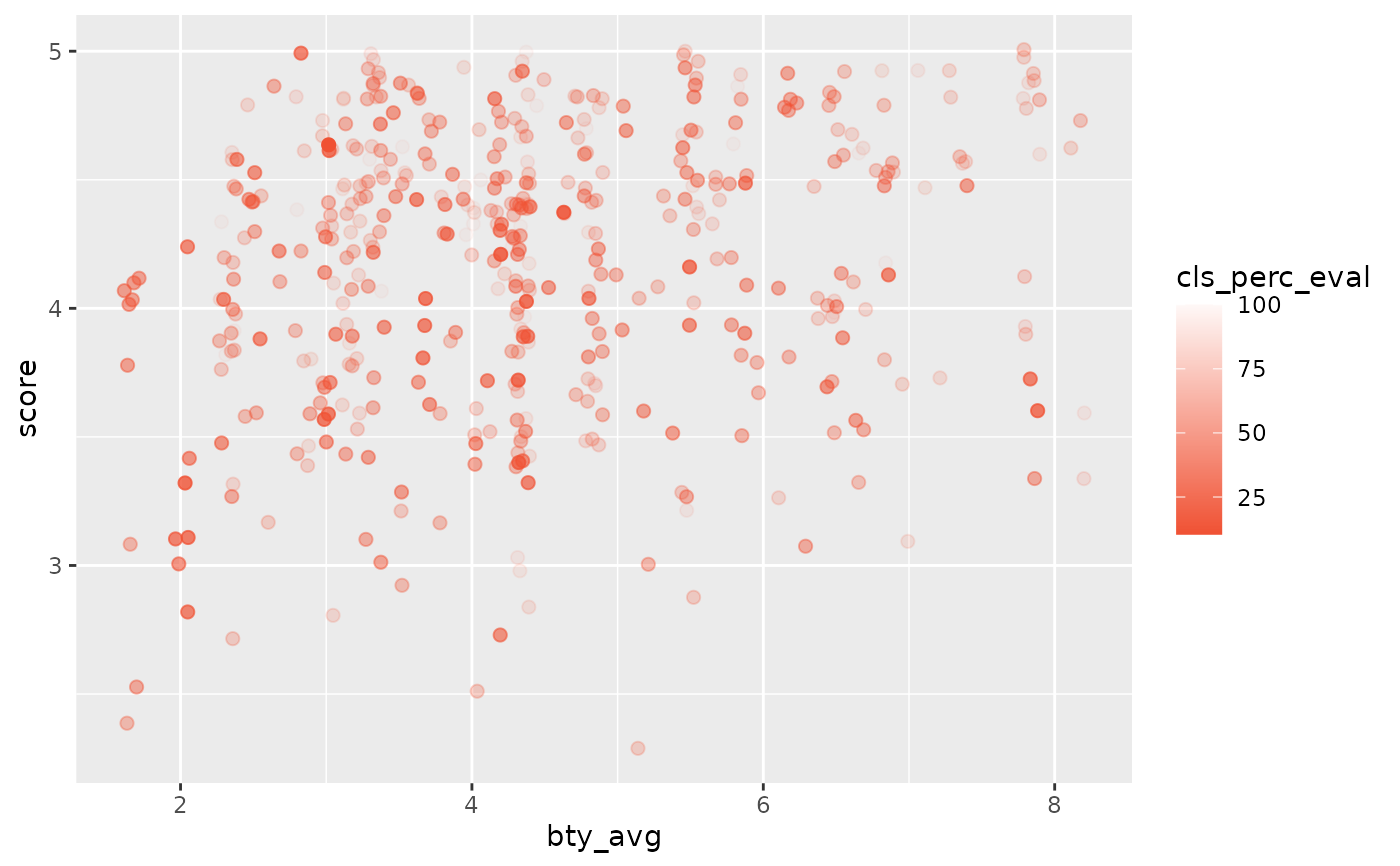Color scale constructor for OpenIntro IMS colors

## Usage

scale_color_openintro(palette = "main", discrete = TRUE, reverse = FALSE, ...)

## Arguments

palette

Character name of palette in openintro_palettes

discrete

Boolean indicating whether color aesthetic is discrete or not

reverse

Boolean indicating whether the palette should be reversed

...

Additional arguments passed to ggplot2::discrete_scale() or ggplot2::scale_color_gradientn(), used respectively when discrete is TRUE or FALSE

## Examples


library(ggplot2)

# Categorical variable with three levels
ggplot(evals, aes(
x = bty_avg, y = score,
color = rank, shape = rank
)) +
geom_jitter(size = 2, alpha = 0.6) +
scale_color_openintro("three")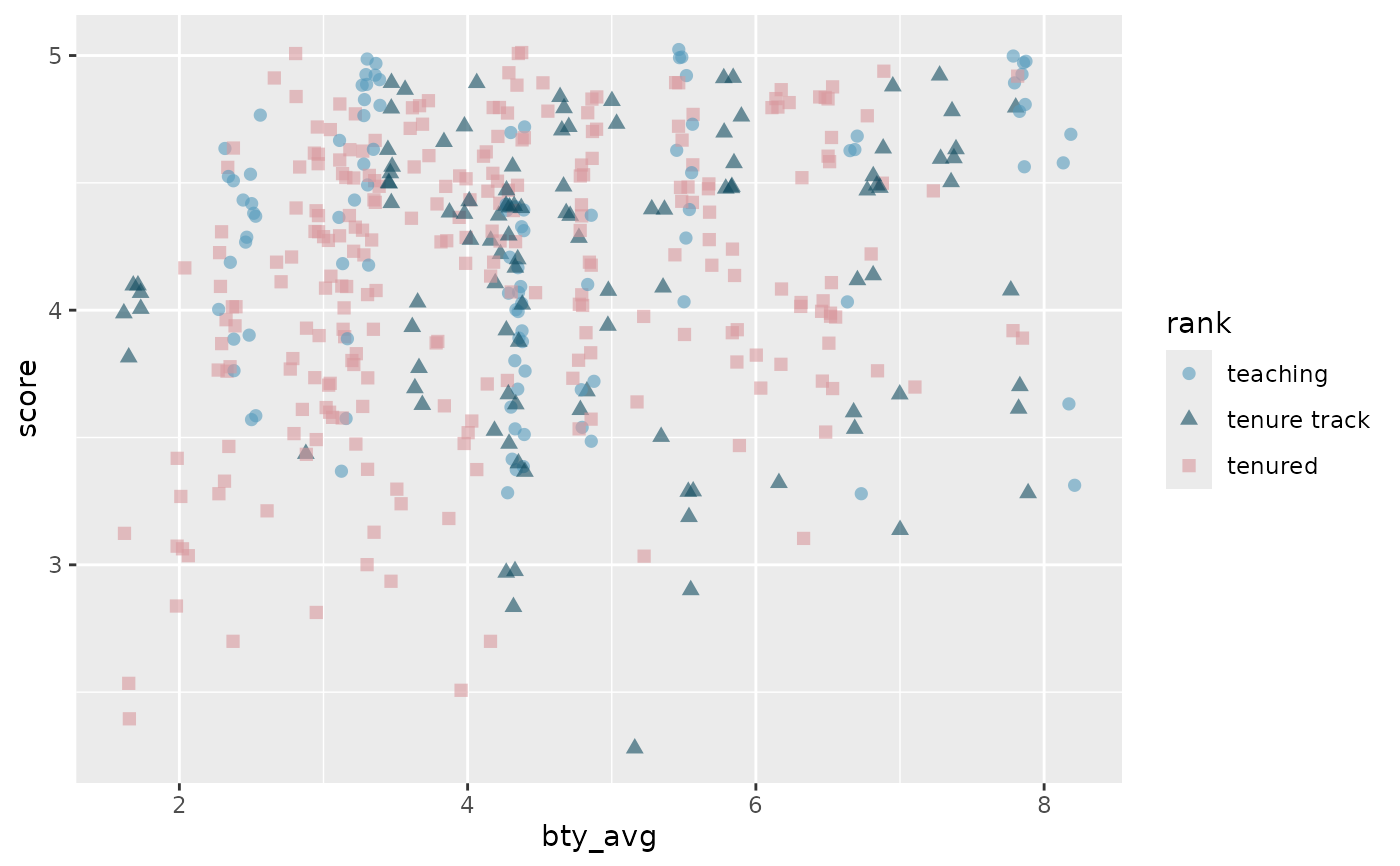# Categorical variable with two levels
ggplot(evals, aes(
x = bty_avg, y = score,
color = language, shape = language
)) +
geom_jitter(size = 2, alpha = 0.6) +
scale_color_openintro("two")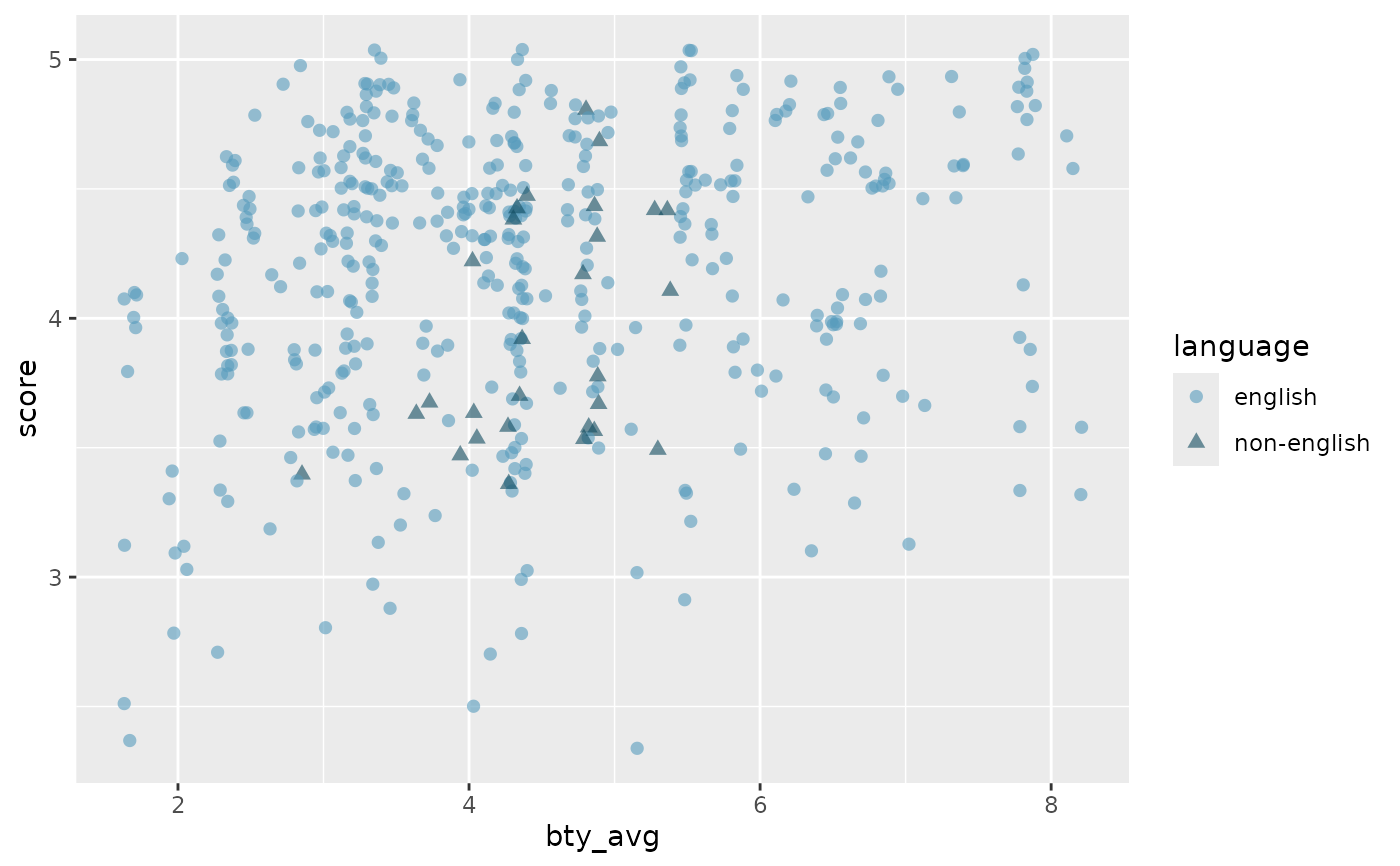# Continuous variable
# Generates a palette, but not recommended
ggplot(evals, aes(
x = bty_avg, y = score,
color = score
)) +
geom_jitter(size = 2, alpha = 0.8) +
scale_color_openintro(discrete = FALSE)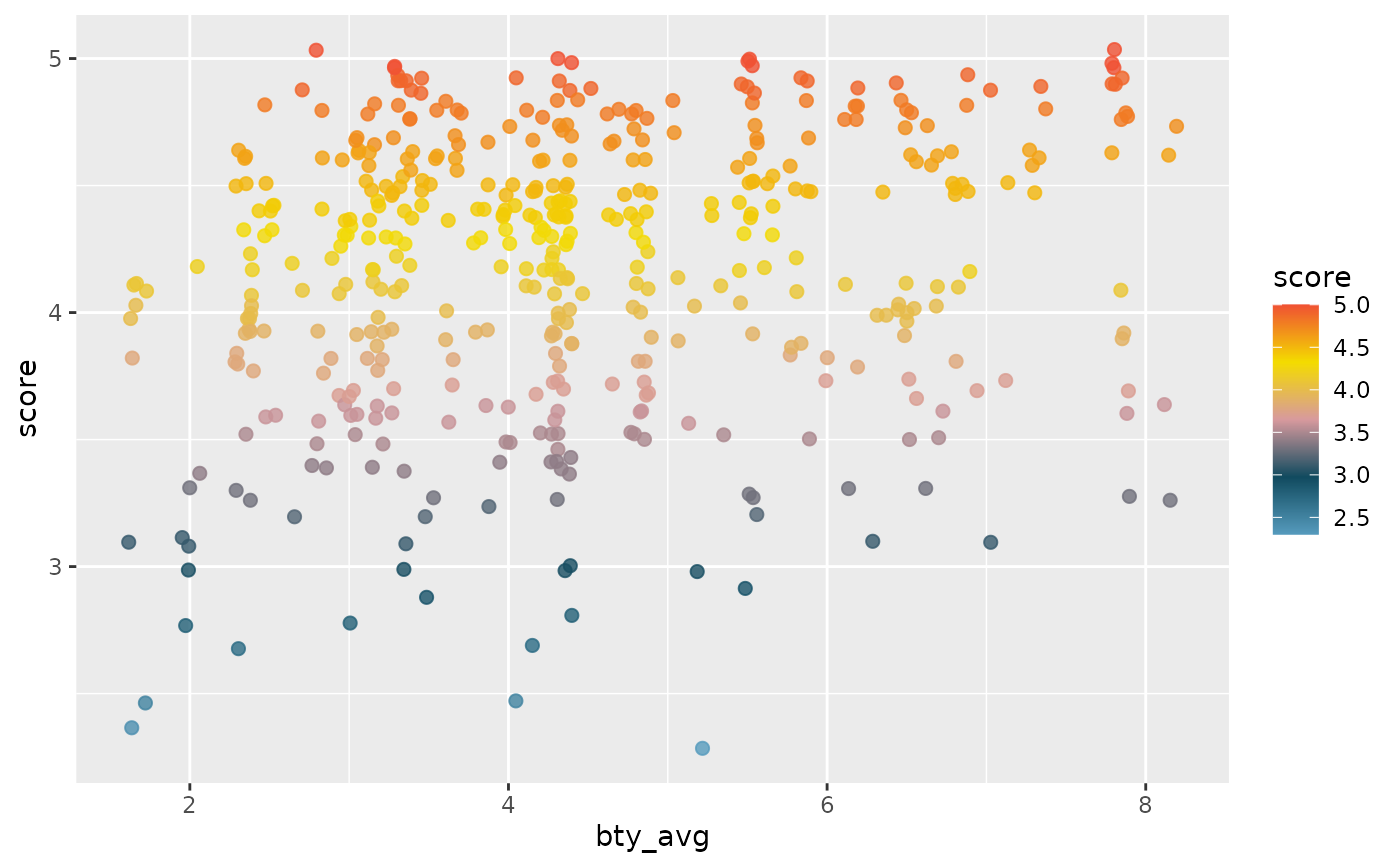# For continous palettes
ggplot(evals, aes(
x = bty_avg, y = score,
color = score
)) +
geom_jitter(size = 2) +
scale_color_gradient(low = IMSCOL["blue", "full"], high = IMSCOL["blue", "f6"])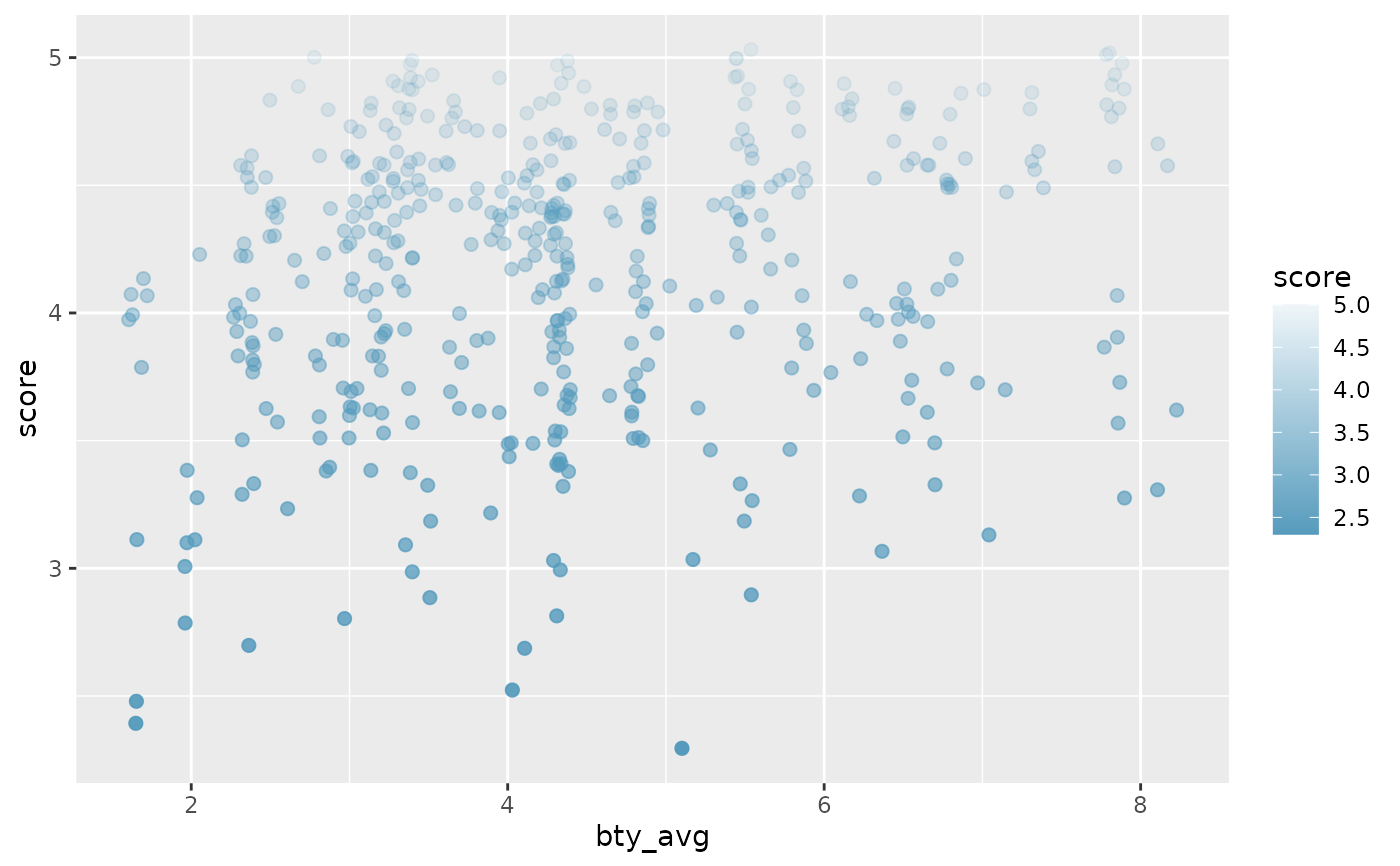ggplot(evals, aes(
x = bty_avg, y = score,
color = cls_perc_eval
)) +
geom_jitter(size = 2) +
scale_color_gradient(low = COL["red", "full"], high = COL["red", "f8"])